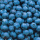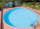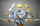# Fraction calculator

The calculator performs basic and advanced operations with fractions, expressions with fractions combined with integers, decimals, and mixed numbers. It also shows detailed step-by-step information about the fraction calculation procedure. Solve problems with two, three, or more fractions and numbers in one expression.

## Result:

### (42/5) : (11/5) = 11/3 = 3 2/3 ≅ 3.6666667

Spelled result in words is eleven thirds (or three and two thirds).

### How do you solve fractions step by step?

1. Conversion a mixed number 4 2/5 to a improper fraction: 4 2/5 = 4 2/5 = 4 · 5 + 2/5 = 20 + 2/5 = 22/5

To find new numerator:
a) Multiply the whole number 4 by the denominator 5. Whole number 4 equally 4 * 5/5 = 20/5
b) Add the answer from previous step 20 to the numerator 2. New numerator is 20 + 2 = 22
c) Write a previous answer (new numerator 22) over the denominator 5.

Four and two fifths is twenty-two fifths
2. Conversion a mixed number 1 1/5 to a improper fraction: 1 1/5 = 1 1/5 = 1 · 5 + 1/5 = 5 + 1/5 = 6/5

To find new numerator:
a) Multiply the whole number 1 by the denominator 5. Whole number 1 equally 1 * 5/5 = 5/5
b) Add the answer from previous step 5 to the numerator 1. New numerator is 5 + 1 = 6
c) Write a previous answer (new numerator 6) over the denominator 5.

One and one fifth is six fifths
3. Divide: 22/5 : 6/5 = 22/5 · 5/6 = 22 · 5/5 · 6 = 110/30 = 10 · 11 /10 · 3 = 11/3
Dividing two fractions is the same as multiplying the first fraction by the reciprocal value of the second fraction. The first sub-step is to find the reciprocal (reverse the numerator and denominator, reciprocal of 6/5 is 5/6) of the second fraction. Next, multiply the two numerators. Then, multiply the two denominators. In the next intermediate step, , cancel by a common factor of 10 gives 11/3.
In words - twenty-two fifths divided by six fifths = eleven thirds.

#### Rules for expressions with fractions:

Fractions - use the slash “/” between the numerator and denominator, i.e., for five-hundredths, enter 5/100. If you are using mixed numbers, be sure to leave a single space between the whole and fraction part.
The slash separates the numerator (number above a fraction line) and denominator (number below).

Mixed numerals (mixed fractions or mixed numbers) write as non-zero integer separated by one space and fraction i.e., 1 2/3 (having the same sign). An example of a negative mixed fraction: -5 1/2.
Because slash is both signs for fraction line and division, we recommended use colon (:) as the operator of division fractions i.e., 1/2 : 3.

Decimals (decimal numbers) enter with a decimal point . and they are automatically converted to fractions - i.e. 1.45.

The colon : and slash / is the symbol of division. Can be used to divide mixed numbers 1 2/3 : 4 3/8 or can be used for write complex fractions i.e. 1/2 : 1/3.
An asterisk * or × is the symbol for multiplication.
Plus + is addition, minus sign - is subtraction and ()[] is mathematical parentheses.
The exponentiation/power symbol is ^ - for example: (7/8-4/5)^2 = (7/8-4/5)2

#### Examples:

subtracting fractions: 2/3 - 1/2
multiplying fractions: 7/8 * 3/9
dividing Fractions: 1/2 : 3/4
exponentiation of fraction: 3/5^3
fractional exponents: 16 ^ 1/2
adding fractions and mixed numbers: 8/5 + 6 2/7
dividing integer and fraction: 5 ÷ 1/2
complex fractions: 5/8 : 2 2/3
decimal to fraction: 0.625
Fraction to Decimal: 1/4
Fraction to Percent: 1/8 %
comparing fractions: 1/4 2/3
multiplying a fraction by a whole number: 6 * 3/4
square root of a fraction: sqrt(1/16)
reducing or simplifying the fraction (simplification) - dividing the numerator and denominator of a fraction by the same non-zero number - equivalent fraction: 4/22
expression with brackets: 1/3 * (1/2 - 3 3/8)
compound fraction: 3/4 of 5/7
fractions multiple: 2/3 of 3/5
divide to find the quotient: 3/5 ÷ 2/3

The calculator follows well-known rules for order of operations. The most common mnemonics for remembering this order of operations are:
PEMDAS - Parentheses, Exponents, Multiplication, Division, Addition, Subtraction.
BEDMAS - Brackets, Exponents, Division, Multiplication, Addition, Subtraction
BODMAS - Brackets, Of or Order, Division, Multiplication, Addition, Subtraction.
GEMDAS - Grouping Symbols - brackets (){}, Exponents, Multiplication, Division, Addition, Subtraction.
Be careful, always do multiplication and division before addition and subtraction. Some operators (+ and -) and (* and /) has the same priority and then must evaluate from left to right.

## Fractions in word problems:

• ExpressionsLet k represent an unknown number, express the following expressions: 1. The sum of the number n and two 2. The quotient of the numbers n and nine 3. Twice the number n 4. The difference between nine and the number n 5. Nine less than the number n
• University bubbleYou'll notice that the college up slowly every other high school. In Slovakia/Czech republic a lot of people studying political science, mass media communication, social work, many sorts of management MBA. Calculate how many times more earns clever 25-yea
• Equation - inverseSolve for x: 7: x = 14: 1000
• Quotient and divisionWhat is the quotient of 2/9 and 1/8?
• AlmondsRudi has 4 cups of almonds. His trail mix recipe calls for 2/3 cup of almonds. How many batches of trail mix can he make?
• SallySally is making 15 individual blueberry cheesecakes. How many pounds of blueberries will be in each cheesecake if 5 1/4 pounds of blueberries are divided equally among them?
• DicesWe will throw two dice. What is the probability that the ratio between numbers on first and second dice will be 1:2?
• How many 14How many 1/2 cup serving are in a package of cheese that contains 5 1/4 cups altogether?
• Leaking swimming poolBetween 10 PM and 7:10 AM, the water level in a swimming pool decreased by 5/12 inches . Assuming that the water level decreased at a constant rate, how much did the water level drop each hour?
• BeerAfter three 10° beers consumed in a short time, there is 5.6 g of alcohol in 6 kg adult human blood. How much is it per mille?
• NumbersDetermine the number of all positive integers less than 4183444 if each is divisible by 29, 7, 17. What is its sum?
• Barbara 2Barbara get 6 pizzas to divide equally among 4 people. How much of a pizza can each person have?
• Divide 13Divide. Simplify your answer and write as an improper fraction or whole number. 14÷8/3